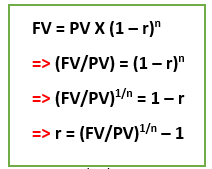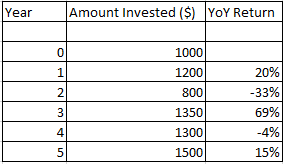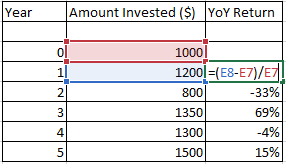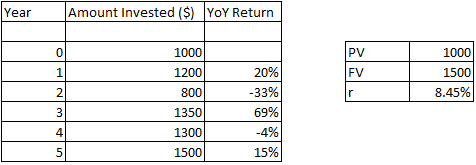# Compound Annual Growth Rate (CAGR)

• Updated on

## What is Compound Annual Growth Rate or CAGR?

Compound Annual Growth Rate is the rate of return that is needed for an investment to grow over a period when the income generated through the amount invested gets re-invested at the end of each year.

Before diving deeper into the CAGR concept, let us understand what compounding means.

## What is compounding?

In simple terms, compounding refers to the addition of interest on interest earned (or charged). It refers to the increasing value of an asset because of the addition of interest on principle as well as the accumulated interest. One can easily relate this concept with a mathematical concept known as compound interest.

Compound interest is computed by subtracting the principal amount from the total amount of principal and interest which we get in future. Mathematically, it is represented as below:## How can one relate CI concept with CAGR formula?Where,

• FV: Final value
• PV: Present value
• r: rate or CAGR
• n: time

In simple terms, we can say that CAGR is the modified version of compound interest.

## Where can you apply CAGR?

As an investor, suppose you look at the P&L statement of a Company and want to know the percentage growth on the equity. Let us assume that in year 1, the Company was able to generate a return on equity (ROE) of 10%, 12% ROE in year 2, and in year 3, an ROE of 15%. As an investor, we would want to look for a representative value at which the ROE increased each year. This value is the CAGR value.

Another application of CAGR could be seen when you check the revenue growth of a Company.

CAGR can be used for personal finance as well. Many investors are interested in mutual funds and want to look at a metric that indicates their respective performances. Let us understand with an example:Calculation of YoY growth on the amount invested using Excel:Calculation of CAGR (r):How was the calculation done on excel?The calculation in excel was done using the formula highlighted above.

Explanation of the above example:

Suppose you have invested \$1,000 in mutual fund M, which provide you \$1,200 after one year. At the start of year 2, you re-invested the amount, but you get \$800. This means you made a loss which is also reflected via the image above. Similarly, in year 3, 4 and 5 you get YoY return of 69%, -4% and 15% respectively.

Overall, if you want to understand how much you return you received after investing in this mutual fund ‘M’, then using the formula you got 8.45% return.

The value which you derived would help you to understand whether it was an excellent decision to invest in this mutual fund or not. You can compare the return of other stock, mutual fund or any financial product and accordingly take a decision.

## The Rule of 72

As an individual, we are always eager to know how much time is needed to double our investment. We have seen long and tedious was to calculate the time where we can see our investment gets doubled. One way is using the formula of compound interest and others using excel. But these consume time in calculation.

Apart from the above methodologies, there is another quick formula which is known as the “Rule of 72”. The formula, which makes the calculation easy, is:

n = 72/r

Here, “r” is the rate while “n” is time.

Let us consider an example.

Let us assume an investor invests \$1,000 in mutual fund (providing 18% interest per annum), \$1,000 in a savings account (providing 4% interest per annum) and fixed deposit (providing interest of 8% per annum). Then using the “Rule of 72,” the investor can double its investment in:

• A mutual fund in 4 years (calculation: 72/18).
• Saving accounts in 18 years ( calculation: 72/4)
• Fixed deposit in 9 years (calculation: 72/8)

Alternatively, if you know the time in which would want the money to grow double and we want to find the rate, then using this rule we can find the rate as well.

Suppose you want to double \$10,000 in 3 years, then my rate would be:

r =  72/3

=  24%

Interpretation: if you want to double your investment in 3 years, then your investment should grow at 24%.

Application of Rule of 72

Rule of 72 is not limited to find the time to double your money. You can use the rule in our everyday lives. Suppose you purchase a product which is currently available in \$20. Now if we want to know the time in which the price of this product will get doubled, assuming the inflation rate is 5%, then what is the period. The answer would be 72/5, which is equal to 14.4 years.

##### Top ASX Listed Companies

Definition:
We use cookies to ensure that we give you the best experience on our website. If you continue to use this site we will assume that you are happy with it. OK